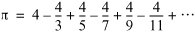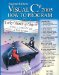# Wrap-Up

### Answers to Self Review Exercises

 6.1 a) for, while. b) after. c) switch. d) continue. e) && (conditional AND) or & (boolean logical AND). f) false. g) static. 6.2 a) False. The default label is optional. If no default action is needed, then there is no need for a default label. b) False. You could terminate the case with other statements, such as a return. c) False. Both of the relational expressions must be true for this entire expression to be true when using the && operator. d) True. e) True. f) False. The switch statement does not provide a mechanism for testing ranges of values, so you must list every value to test in a separate case label. g) True. 6.3 sum = 0; for ( count = 1; count <= 99; count += 2 ) sum += count; double result = Math.Pow( 2.5, 3 ); i = 1; while ( i <= 20 ) { Console.Write( i ); if ( i % 5 == 0 ) Console.WriteLine(); else Console.Write( ' ' ); i++; } for ( i = 1; i <= 20; i++ ) { Console.Write( i ); if ( i % 5 == 0 ) Console.WriteLine(); else Console.Write( ' ' ); } 6.4 Error: The semicolon after the while header causes an infinite loop, and there is a missing left brace for the body of the while statement. Correction: Remove the semicolon and add a { before the loop's body. Error: Using a floating-point number to control a for statement may not work, because floating-point numbers are represented only approximately by most computers. Correction: Use an integer, and perform the proper calculation in order to get the values you desire: ```for ( k = 1; k < 10; k++ ) Console.WriteLine( ( double ) k / 10 ); ``` Error: case 1 cannot fall through into case 2. Correction: Terminate the case in some way, such as adding a break statement at the end of the statements for the first case. Error: An improper relational operator is used in the while repetition-continuation condition. Correction: Use <= rather than <, or change 10 to 11.

### Exercises

6.5

Describe the four basic elements of counter-controlled repetition.

6.6

Compare and contrast the while and for repetition statements.

6.7

Discuss a situation in which it would be more appropriate to use a do...while statement than a while statement. Explain why.

6.8

Compare and contrast the break and continue statements.

6.9

Find and correct the error(s) in each of the following segments of code:

1. For ( i = 100, i >= 1, i++ ) Console.WriteLine( i );
2. The following code should print whether integer value is odd or even:

```
```
switch ( value % 2 ) { case 0: Console.WriteLine( "Even integer" ); case 1: Console.WriteLine( "Odd integer" ); }
3. The following code should output the odd integers from 19 to 1:

``` for ( int i = 19; i >= 1; i += 2 )
Console.WriteLine( i );
```
4. The following code should output the even integers from 2 to 100:

``` counter = 2;

do
{
Console.WriteLine( counter );
counter += 2;
} while ( counter < 100 );
```
6.10

What does the following application do?

``` 1 // Exercise 6.10 Solution: Printing.cs
2 using System;
3
4 public class Printing
5 {
6 public static void Main( string[] args )
7 {
8 for ( int i = 1; i <= 10; i++ )
9 {
10 for ( int j = 1; j <= 5; j++ )
11 Console.Write( '@' );
12
13 Console.WriteLine();
14 } // end outer for
15 } // end Main
16 } // end class Printing
```
6.11

Write an application that finds the smallest of several integers. Assume that the first value read specifies the number of values to input from the user.

6.12

Write an application that calculates the product of the odd integers from 1 to 7.

6.13

Factorials are used frequently in probability problems. The factorial of a positive integer n (written n! and pronounced "n factorial") is equal to the product of the positive integers from 1 to n. Write an application that evaluates the factorials of the integers from 1 to 5. Display the results in tabular format. What difficulty might prevent you from calculating the factorial of 20?

6.14

Modify the compound-interest application of Fig. 6.6 to repeat its steps for interest rates of 5, 6, 7, 8, 9 and 10%. Use a for loop to vary the interest rate.

6.15

Write an application that displays the following patterns separately, one below the other. Use for loops to generate the patterns. All asterisks (*) should be printed by a single statement of the form Console.Write( '*' ); which causes the asterisks to print side by side. A statement of the form Console.WriteLine(); can be used to move to the next line. A statement of the form Console.Write( ' ' ); can be used to display a space for the last two patterns. There should be no other output statements in the application. [Hint: The last two patterns require that each line begin with an appropriate number of blank spaces.]

```(a) (b) (c) (d)

* ********** ********** *
** ********* ********* **
*** ******** ******** ***
**** ******* ******* ****
***** ****** ****** *****
****** ***** ***** ******
******* **** **** *******
******** *** *** ********
********* ** ** *********
********** * * **********
```
6.16

One interesting application of computers is to display graphs and bar charts. Write an application that reads three numbers between 1 and 30. For each number that is read, your application should display the same number of adjacent asterisks. For example, if your application reads the number 7, it should display *******.

6.17

A Web site sells three products whose retail prices are as follows: product 1, \$2.98; product 2, \$4.50; and product 3, \$9.98. Write an application that reads a series of pairs of numbers as follows:

1. product number
2. quantity sold

Your application should use a switch statement to determine the retail price for each product. It should calculate and display the total retail value of all products sold. Use a sentinel-controlled loop to determine when the application should stop looping and display the final results.

6.18

In the future, you may work with other programming languages that do not have a type like decimal which supports precise monetary calculations. In those languages, you should perform such calculations using integers. Modify the application in Fig. 6.6 to use only integers to calculate the compound interest. Treat all monetary amounts as integral numbers of pennies. Then break the result into its dollars and cents portions by using the division and remainder operations, respectively. Insert a period between the dollars and the cents portions when you display the results.

6.19

Assume that i = 1, j = 2, k = 3 and m = 2. What does each of the following statements print?

1. Console.WriteLine( i == 1 );
2. Console.WriteLine( j == 3 );
3. Console.WriteLine( ( i >= 1 ) && ( j < 4 ) );
4. Console.WriteLine( ( m <= 99 ) & ( k < m ) );
5. Console.WriteLine( ( j >= i ) || ( k == m ) );
6. Console.WriteLine( ( k + m < j ) | ( 3 - j >= k ) );
7. Console.WriteLine( !( k > m ) );
6.20

Calculate the value of p from the infinite seriesPrint a table that shows the value of p approximated by computing one term of this series, by two terms, by three terms, and so on. How many terms of this series do you have to use before you first get 3.14? 3.141? 3.1415? 3.14159?

6.21

(Pythagorean Triples) A right triangle can have sides whose lengths are all integers. The set of three integer values for the lengths of the sides of a right triangle is called a Pythagorean triple. The lengths of the three sides must satisfy the relationship that the sum of the squares of two of the sides is equal to the square of the hypotenuse. Write an application to find all Pythagorean triples for side1, side2 and the hypotenuse, all no larger than 500. Use a triple-nested for loop that tries all possibilities. This method is an example of "brute-force" computing. You will learn in more advanced computer science courses that there are large numbers of interesting problems for which there is no known algorithmic approach other than using sheer brute force.

6.22

Modify Exercise 6.15 to combine your code from the four separate triangles of asterisks such that all four patterns print side by side. Make clever use of nested for loops.

6.23

Write an application that prints the following diamond shape. You may use output statements that print a single asterisk (*), a single space or a single newline character. Maximize your use of repetition (with nested for statements) and minimize the number of output statements.

 ``` * *** ***** ******* ********* ******* ***** *** * ```
6.24

Modify the application you wrote in Exercise 6.23 to read an odd number in the range 1 to 19 to specify the number of rows in the diamond. Your application should then display a diamond of the appropriate size.

6.25

A criticism of the break statement and the continue statement is that each is unstructured. Actually, break statements and continue statements can always be replaced by structured statements, although doing so can be awkward. Describe in general how you would remove any break statement from a loop in an application and replace that statement with some structured equivalent. [Hint: The break statement exits a loop from the body of the loop. The other way to exit is by failing the loop-continuation test. Consider using in the loop-continuation test a second test that indicates "early exit because of a 'break' condition."] Use the technique you develop here to remove the break statement from the application in Fig. 6.12.

6.26

What does the following code segment do?

```for ( int i = 1; i <= 5; i++ )
{
for ( int j = 1; j <= 3; j++ )
{
for ( int k = 1; k <= 4; k++ )
Console.Write( '*' );

Console.WriteLine();
} // end inner for

Console.WriteLine();
} // end outer for
```
6.27

Describe in general how you would remove any continue statement from a loop in an application and replace it with some structured equivalent. Use the technique you develop here to remove the continue statement from the application in Fig. 6.13.Visual C# 2005 How to Program (2nd Edition)
ISBN: 0131525239
EAN: 2147483647
Year: 2004
Pages: 600

Similar book on Amazon